#Function Repository Resource:

# NInverseFourierCosTransform

Find a numerical approximation for an inverse Fourier cosine transform

Contributed by: Wolfram Research
 ResourceFunction["NInverseFourierCosTransform"][expr,ω,t] gives a numerical approximation to the inverse Fourier cosine transform of expr evaluated at the numerical value t, where expr is a function of ω.

## Details and Options

The numerical approximation to the inverse Fourier cosine transform of expr is by default defined to be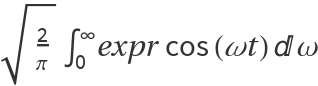.
Different choices for the definition of the inverse Fourier cosine transform can be specified using the option FourierParameters.
With the setting FourierParameters{a,b}, the inverse Fourier cosine transform computed by ResourceFunction["NInverseFourierCosTransform"] is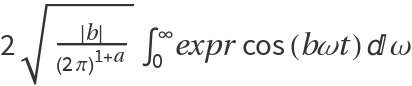.
The parameter b in the setting FourierParameters{a,b} must be numeric.
In addition to the option FourierParameters, ResourceFunction["NInverseFourierCosTransform"] can also accept the options available to NIntegrate. These options are passed directly to NIntegrate.

## Examples

### Basic Examples

Numerical approximation for an inverse Fourier cosine transform:

 In:=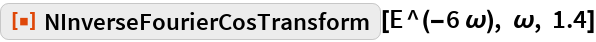Out=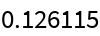Compare with the answer from symbolic evaluation:

 In:=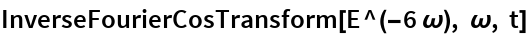Out=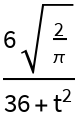In:=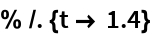Out=## Requirements

Wolfram Language 11.3 (March 2018) or above